## Agricultural Science Digest

• Chief EditorArvind kumar

• Print ISSN 0253-150X

• Online ISSN 0976-0547

• NAAS Rating 4.75

• SJR 0.156

Frequency :
Bi-monthly (February, April, June, August, October and December)
Indexing Services :
BIOSIS Preview, Biological Abstracts, Elsevier (Scopus and Embase), AGRICOLA, Google Scholar, CrossRef, CAB Abstracting Journals, Chemical Abstracts, Indian Science Abstracts, EBSCO Indexing Services, Index Copernicus
Agricultural Science Digest, volume 43 issue 2 (april 2023) : 157-163

# Validation of Models for Simulating the Soil Moisture Characteristics

P.H. Rank1,*, B. Vishnu2
1Department of Irrigation and Drainage Engineering, Junagadh Agricultural University, Junagadh-362 001, Gujarat, India.
2Regional Agricultural Research Station, Kerala Agricultural University, Kumarakom-686 563, Kerala, India.
• Submitted16-10-2021|

• Accepted22-06-2022|

• First Online 16-07-2022|

Cite article:- Rank P.H., Vishnu B. (2023). Validation of Models for Simulating the Soil Moisture Characteristics . Agricultural Science Digest. 43(2): 157-163. doi: 10.18805/ag.D-5517.
Background: The determination of the soil moisture characteristic is a tedious, lengthy and costly laboratory methods. However, for the specific soil texture, once it is known, the soil moisture at various tension can be simulated through modelling approach which eliminates the requirements of performing the experiments every time. Therefore, the attempt was made for the determinations of the soil moisture characteristics during the year-2019 and validating the various models’ using these empirical data.

Methods: The present research covers the experimentation during the year 2019 for determination of the soil moisture characteristics and fitting the obtained empirical data to mathematical frames of the various models. The moisture characteristics was obtained by measuring different moisture content values at different suction values through two experiments viz. wetting cycle (Absorption phase) and drying cycle (Desorption phase). The desorption curve was obtained by using the pressure plate apparatus and the wetting curve was measured through the capillary rise open tube method.

Result: The saturation, field capacity, permanent wilting, residual moisture content, void ratio and saturated hydraulic conductivity were found as 43.5%v/v, 25.88%v/v, 12.05%v/v, 8.1%v/v. 0.77 and 0.2808 m/day respectively. Among the fitted models, the van Genuchten model was found best fit to observe data to simulate the desorption and sorption phase of the soil moisture retention characteristics. The soil moisture retention characteristics of the sorption and desorption phases indicated a hysteresis of approximately two log cycles.
The optimal crop yield and water use efficiency can be achieved through sound irrigation scheduling by imposing the optimal moisture stress during different growth stages (Rank and Satasiya, 2022; Rank et al., 2022). The deficit irrigation with optimal moisture stress can improve the root aeration during irrigation interval in case surface irrigation and even during irrigation in case of pulse drip method (Rank and Vishnu, 2021a). The automation of pulse irrigation was performed well by Rank and Vishnu, (2019). The Wetting pattern study depicts the root aeration in the soil (Rank et al., 2019). The moisture stress requires the soil moisture retention characteristics. The soil moisture characteristic represents the relationship between the soil moisture tension and corresponding moisture content. It was also known that soil water retention curve experiences hysteresis effect between wetting cycle and drying cycle. The important practical applications of soil water retention curve made itself to be a major research issue for the unsaturated conditions. The water retention curve depicts the characteristics of soil which is also termed as soil water characteristic curve (SWCC). The water retention curve is the relationship between the volumetric water content and matric suction. Different wetting and drying curves could be distinguished for the hysteretic effect of water filling the pores of soil as well as draining from the pores. Greater the clay content, the greater the water content at any particular suction and more gradual the slope of the curve. Fredlund and Xing (1994) proposed a general equation for predicting soil moisture characteristic curve which was based on assumption that the soil moisture characteristic is dependent on pore size distribution of the soil. Once a reasonable estimate of the soil-water characteristic curve is obtained, satisfactory predictions of the shear strength function can be made for the unsaturated soil. The degree of saturation corresponds to a particular suction could be defined for the soil and thus becomes measure of the pore size distribution of the soil. The one of the issues in modelling SWCC is that for a given soil, an infinite number of scanning curves exists, resulting from different wetting and drying hysteresis and soil states (Li, 2005). The most influential state variable in this regard is the soil density. The past research on pulse irrigation was reviewed extensively and concluded that wetting and drying characteristics are affecting the soil moisture regimes in the crop root zone (Rank and Vishnu, 2021b). Hysteresis loop generated from alternate drying and wetting processes expressively varies from monotonic drying and wetting curves. Besides a number of existing hysteresis models, there are also many experiential models that have been anticipated. There is no physical base for these models, but because of their straight forwardness, these models have been used in some applications such as Scaling-down model, Curve-fitting model etc. (Fredlund and Xing, 1994).

The soil moisture characteristics differs with soil texture, biochemical as well as physic-chemical properties of soil which varies from region to region. Therefore, the nature of best fit model and its parameters which can simulate more closely the observed SWCC can also vary.
The physio-chemical properties of the soil like moisture content, bulk density and dry density, bulk density, dry density, soil texture, liquid limit and plastic limit, hydraulic conductivity, specific gravity, EC and pH were determined adopting the standard methodology and instruments at the KCAET Research Farm, KAU, Tavnur, Kerala.

Soil moisture characteristic

It represents the relationship between the soil suction and corresponding moisture content of soil. Tensiometric irrigation scheduling could be done by the soil moisture characteristic curves. These curves are hydraulic properties related to size and pores spaces that are strongly affected by soil texture and structure. The moisture characteristics curve was obtained by measuring different moisture content values at different suction values. It was also known that soil water retention curve experiences hysteresis effect when it comes to wetting cycle from the drying cycle. The drying curve was obtained by using the pressure plate apparatus manufactured by Soil Moisture Equipment Cooperation (USA). The wetting cycle was measured through the capillary rise open tube method (Yang et al., 2004).

Absorption process during wetting phase

The soil retention curve for wetting cycle can be obtained by capillary rise open tube. The capillary rise tube was filled with the undisturbed soil. The tube was placed in the soil fully through hammer to get undisturbed soil samples. The tubes were then oven dried. These tubes were placed into the tray and water table was maintained in the tray. The top of the tubes was covered with cloth to prevent evaporation. Water in the tray starts to move in to the soil through capillary actions as soon as it was placed into the tray. The capillary tube was reached to equilibrium within 2 weeks. Water table in tray was maintained constant. After getting equilibrium, water level was found constant. Then soil samples were taken out from various levels. The samples were oven dried to determine moisture content. The height of the soil specimen above the water level was assumed equal to capillary or negative pore-water pressure. This magnitude of negative pore-water pressure head is equal to the matric suction head at that point. Air pressure in this tube was at atmospheric pressure. The plot of water content and versus matric suction gives the wetting soil moisture characteristic curve of soil (Yang et al., 2004). These capillary tubes were kept until the equilibrium between soil capillary action or upward movement. The moisture content at different heights were measured and tabulated to prepare wetting cycle of SWCC.

Desorption process during drying phase

The soil retention curve for drying cycle can be obtained by setting the required pressure in pressure plate apparatus and finding respective retained moisture content in soil. The basic principle of this apparatus is to apply the different matric tensions to the saturated sample placed between the porous plates. For determining the moisture characteristic curve, this apparatus was used as a standard technique. The samples were first saturated and were placed on a porous ceramic plate inside the pressure chamber. The ceramic plate was maintained at atmospheric pressure while samples were pressurized and thus subsequent flow occurs through saturated ceramic plate from the samples. Once the soil samples reach equilibrium with the imposed pressure, the flow ceases. The retained moisture content at set suction pressure of 0, 0.1, 0.33, 1.0, 3.0, 5.0 and 15.0 bar were determined using gravimetric method. Then the measured moisture content at corresponding suction were plotted on graph. The soil water retention curve was drawn using that observed values. The soil moisture characteristics is basically used for the determination of field capacity and permanent wilting point moisture values. According to theory, the field capacity is generally attained at 1/10 to 1/3 bar suction pressure whereas the permanent wilting point is attained at 15 bar pressure.

Hysteresis effect of soil moisture characteristic

Hysteresis in the SWCC refers to the non-unique relationship between the soil’s matric suction and its water content, whereby the soil can have two different water contents at the same matric suction value, depending on the preceding sequence of wetting and drying (Bashir et al., 2015). Various factors can be the reasons of such hysteresis effects. The different contact angles in the advancing and receding soil-air interface menisci, variable and irregular cross-sections of the pores and the difference in entrapped air volume at different matric suction values are some of the factors causing hysteresis effect.

Simulation models for soil moisture characteristics

The traditional ways to determine the moisture retention curves involves many methods with and without considering the hysteresis effect. The model was developed by different researchers and were having different characteristics, some were accurate for particular soil whereas some were accurate for all type of soils. These models either can be based on soil texture or basic index soil properties. Some of the models were discussed and validated in the present study.

van Genuchten 1980 model

The simulation of fluid flow in unsaturated condition was became very popular and many models were based on derivation of closed form analytical expression of hydraulic conductivity models. From the information of soil water characteristic curve and saturated conductivity Mualem, 1976 derived a model predicting hydraulic conductivity. Maulem’s equation lead to a simple integral formula for unsaturated hydraulic conductivity that gave an opportunity to derive the closed form analytical expressions and providing suitable equations for Soil water retention curve. The soil moisture content as a function of pressure head is given by: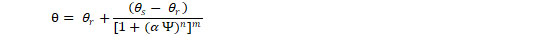Where,
θ= Volumetric water content (cm3 cm-3).
θr= The residual water content.
θs= The saturated water content.
α= (kPa-1), n and m [m= 1- (1/n)] are empirical parameters.
ψ = Matric potential (kPa).

As per the above equation four independent variables θs, θr, α and n are to be estimated from observed soil moisture characteristic data. Saturated water content θs and θr can be easily obtained by experiment in laboratory. Estimation of the van Genuchten model’s basic equations parameters were given by Ghanbarian et al. (2010). The value of a is determined from the table and m, n were found out through :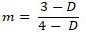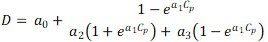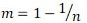Where,
D = Fractal dimension of soil water retention curve.
Cp = Soil clay percentage.
a0, a1, a2 and a3 = Constant coefficients varies with types of soils.

Ghanbarian model itself derives the soil moisture characteristic curve with his derived equation. Maulem’s equation lead to a simple integral formula for unsaturated hydraulic conductivity that gave an opportunity to derive the closed form analytical expressions and providing suitable equations for Soil water retention curve.
Maulem’s derived following equation: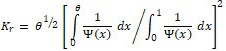Where,
ψ = Pressure head given as a function of volumetric water content.

Burdine, 1953 model also gave similar results as above for Maulem, 1976 model. The equation given by (Burdine, 1953):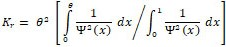The analysis is same as before. Primary tests indicate that Burdine based equations were in most in lesser agreement with experimental data than the Maulem based expressions. The prediction based on Maulem’s theory that was basically by means of numerical approximations, were generally more accurate than those based on Burdine’s various forms.

Fredlund and Xing 1994 model

To describe the general form of predictive soil water retention curve over entire suction range, volumetric water content is referenced as zero moisture content. Volumetric water content and soil moisture suction relationship can be given by: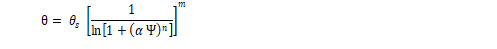According to above equation when suction is zero, θ becomes equal to θs and when suction goes, infinity θ becomes zero. Degree of saturation can also be used for curve fitting. Nevertheless, experimental data have shown that at zero moisture content the suction of soil reaches maximum value of approximately 106 kPa (Fredlund and Xing, 1994). The upper limit can be given to above equation as following: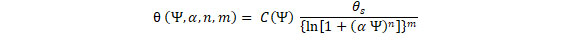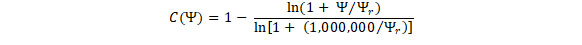Where,
ψr= The suction corresponding to the residual water content θr.

Parameters of Fredlund and Xing model were determined from Zapata et al., 2000 and Perera et al., 2005 models, from which parameters were simulated. Both the models are soil moisture characteristic models based on soil index properties.

The soil moisture retention curve given by Zapata et al. (2000) is following: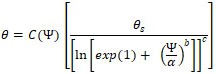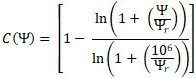Where,
θ= The volumetric water content (cm3 cm-3).
θs= The saturated water content, a, b, c.
ψr= Empirical parameters.
ψ= The matric potential (kPa).
C(ψ)= Correction factor which is a function of matric suction. These empirical parameters are calculated differently based on fine grained and coarse-grained soils.

Fine grained soil parameters

When Plasticity Index (wPI) > 0,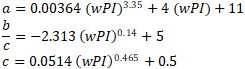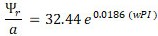Coarse grained soil parameters

When Plasticity Index (wPI) = 0,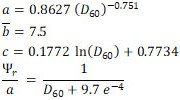Where,
D60 = Grain diameter corresponding to 60% of weight passing.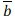= Average value of fitting parameter.

The soil moisture retention curve given by Perera et al. (2005) is following: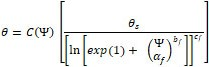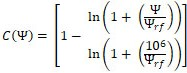Where,
θ = Volumetric water content (cm3 cm-3).
θs = Saturated water content.
af =  Fitting parameter which is primarily a function of the air entry value of the soil.
bf =  Fitting parameter, which is primarily a function of the rate of water extraction from the soil, once the air entry value has been exceeded.
cf = Fitting parameter, which is primarily a function of residual  water content.
Ψrf = Fitting parameter, which is primarily a function of the suction at which residual water content occurs.
Ψ = Matric potential (kPa).

These empirical parameters are calculated differently based on fine grained and coarse grained soils:

Fine grained soil parameters

When Plasticity Index (wPI) > 0.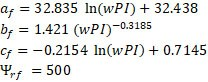Coarse grained soil parameters

When Plasticity Index (wPI) = 0, for non-plastic soil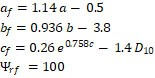The input values for non-plastic soils are D10, D20, D30, D60, D90, silt content and voids ratio whereas for plastic soil only plasticity index and voids ratio data are needed.

Gardner 1958 model

Gardner proposed an equation for the permeability function. The equation simulates the Soil water retention curve and can be visualized by van Genuchten equation.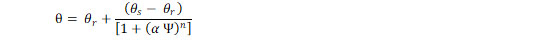Where,
θ= Volumetric water content (cm3 cm-3).
θr= Residual water content.
θs= Saturated water content.
α (kPa-1) and n = Empirical parameters.
The observed data during absorption and desorption processes were fitted to models. The model efficiencies were computed using simulated and observed data. The Basic index properties of soil were input for the models and the output of model was compared with observed data. The different models fitted for input data were van Genuchten, Garder, Fredlund and Xing1 and Fredlund and Xing2.

van Genuchten et al., (1980) model

The input data as Clay Content (Cp), Voids Ratio (e) and Saturated Hydraulic Conductivity (Ks), thus gives the value of qr, qs, a, n and m for drying curve. For wetting curve above parameters were determined from Sigma Plot software. The equation to measure Nash-Sutcliffe model efficiency (NSE) is as follow :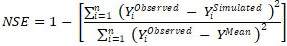Where,
YiObserved = ith observation to be evaluated,
YiSimulated = ith simulated observation to be evaluated.
YMean = Mean of observed data to be evaluated.
n = Total number of observations.

The observed and simulated data of soil moisture retention for various sanction during absorption and desorption processes are depicted in Fig 1.

It can be seen that observed data are closely hugged the model simulated curve indicating good predictive model efficiency. Also, the Nash-Sutcliffe model efficiency (NSE) was found as 0.9874 for drying curve and 0.9226 for wetting curve for van Genuchten model. The R2 value was found 0.9858 in drying and 0.9456 in wetting curve, shows that drying curve predicted data are more closed to observe ones as compared to that of wetting processes (Fig 2).Fig 1: Comparison of observed and simulated moisture content during drying and wetting by van Genuchten et al., (1980) model.Fig 2: Goodness of fit for observed and simulated moisture content during drying and wetting by van Genuchten et al., (1980) model.

Gardner, (1958) model

The model parameters for Gardner are taken same as van Genuchten except parameter-m as Gardner model doesn’t consider it. The observed data and simulated curve by Gardner model are depicted in Fig 3. It shows that observed data and simulated data by model did not matched.

Also, the Nash-Sutcliffe model efficiency (NSE) was found very low as -0.042 for drying curve and 0.026 for wetting curve for Gardner model. The R2 value was found 0.6462 in drying and 0.7478 in wetting curve, shows that neither drying curve predicted data nor wetting curve predicted data are more closed to observed ones (Fig 4).Fig 3: Comparison of observed and simulated moisture content during drying and wetting by Gardner, (1958) model.Fig 4: Goodness of fit for observed and simulated moisture content during drying and wetting by Gardner, (1958) model.

Fredlund and Xing (1) model

The model input parameters were D10, D20, D30, D60, D90, Voids ratio (e), silt content (P200), thus gives the value of qr, qs, a, n and m for drying curve. For wetting curve above parameters were determined from Sigma Plot software. The observed and simulated data by model during absorption and sorption processes are depicted in Fig 5. It can be seen that the observed data are moderately matched with simulated curve by model. In fact, it was clearly reflected in Fig 6 and values of model efficiency and goodness of fit. Nash-Sutcliffe model efficiency (NSE) was found 0.7501 for drying curve and 0.9193 for wetting curve for Fredlund and Xing (1) model. The R2 value was found 0.8627 in drying and 0.9343 in wetting curve, shows that wetting curve predicted data are more closed to observed ones.Fig 5: Comparison of observed and simulated moisture content during drying and wetting by Fredlund and Xing, 1994 (1) model.Fig 6: Goodness of fit for observed and simulated moisture content during drying and wetting by Fredlund and Xing, 1994 (1) model.

Fredlund and Xing 1994 model (2)

The model parameters are taken same as Fredlund and Xing, 1994 (1) model except the qr was eliminated from Fredlund and Xing 1994 model (1) in the modified Fredlund and Xing (2) model. The observed data of soil moisture retention at various suction are compared with simulated curve by model in Fig 7. It can be seen that the observed data deviate more from simulated curve by Fredlund and Xing 1994 model (2) as competed to that of Fredlund and Xing 1994 model (1) as can be reflected in Fig 8 too. Also, the Nash-Sutcliffe model efficiency (NSE) was found very low as 0.062 for drying curve and 0.6128 for wetting curve for Fredlund and Xing (2) model. The R2 value was found 0.6945 in drying and 0.8775 in wetting curve, shows that wetting curve predicted data are more closed to observed ones.Fig 7: Comparison of observed and simulated moisture content during drying and wetting by Fredlund and Xing, 1994 (2) model.Fig 8: Goodness of fit for observed and simulated moisture content during drying and wetting by Fredlund and Xing, 1994 (2) model.

The soil moisture characteristics during the absorption and sorption phases differs due to hysteresis nature of soil to retain the moisture at various suctions. The simulation of soil moisture retention at various suction by different models differs widely with models as different models have their different mathematical frame taking different parameters. Therefore, its predictive nature as well as simulating efficiency differs with model as discussed by Burdine (1953), Gardner (1958), Mualem (1976), van Genuchten (1980), Fredlund and Xing (1994), Zapata et al., (2000), Perera et al. (2005) and Ghanbarian et al., (2010).

The observed data of soil moisture retention at various suction during the absorption and desorption process were obtained through experimentation. The observed data were used to fit the simulation models. Nash-Sutcliffe model efficiency (NSE) and Goodness of fit were computed for model comparisons. The outcomes of the present investigation could yield the following conclusions after churning thoroughly the results.
The texture class of the experimental field soil was found sandy loam having proportion of clay, silt and sand particles as 7.32%, 36.59% and 56.10 % respectively. The volumetric soil moisture content at saturation, field capacity, permanent wilting and residual moisture content were 43.5%, 25.88%, 12.05% and 8.1%. The void ratio and saturated hydraulic conductivity of the soil was found as 0.77 and 0.2808 m/day respectively.
a) The soil moisture retention characteristics of the sorption and desorption phases indicated a hysteresis of approximately two log cycles.
b) Among the four models, the van Genuchten model was found best fit to observe data as below with Nash-Sutcliffe model efficiency of 98.75% (R2=0.986) for desorption and 92.26% (R2=0.946) for sorption to simulate the desorption and sorption phase of the soil moisture retention characteristics.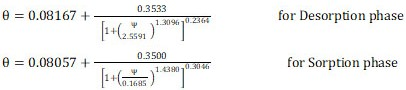Where,
θr, θs and θ = Residual moisture content, saturated soil moisture content and moisture content (cc/cc) at any suction pressure ψ (kPa).
The authors are grateful to the academic and supporting staff of Kellapaji College of Agricultural Engineering and Technology, Tavnur for guiding support and authorities of Kerala Agricultural University, Trissur for financial supports for the present research.
None.

View all (0)